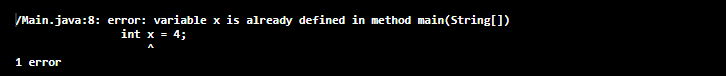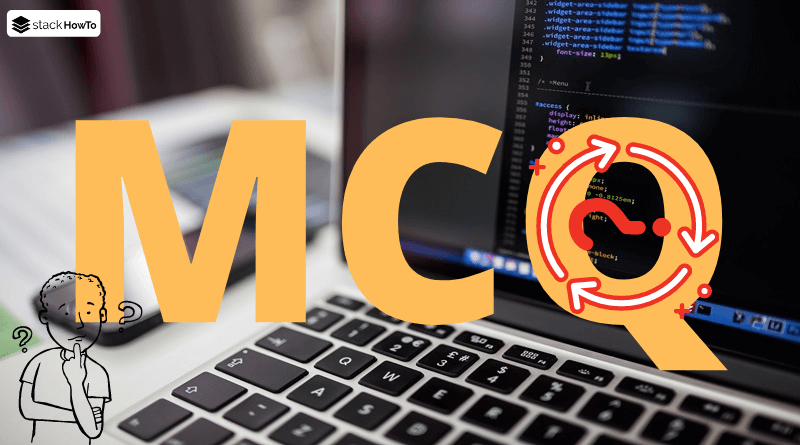MCQ

# Java MCQ – Classes and Objects

This collection of Java Multiple Choice Questions and Answers (MCQs): Quizzes & Practice Tests with Answer focuses on “Classes and Objects”.

###### 1. What is stored in “obj” in the following line of code?
`MyClass obj;`

A NULL

B Pointer

C Memory address allocated for the object

D Garbage

A
Memory is allocated to an object using the “new” operator. while `MyClass obj;` simply declares a reference to the object, no memory is allocated to it, hence it points to NULL.

###### 2. Which of these keywords is used to create a class?

A class

B struct

C int

D None of the above

A
In Java, we use the keyword “class” to create a class.

###### 3. Which of the following statements is a valid declaration of an object that belongs to “MyClass”?

A `MyClass obj = new MyClass();`

B `MyClass obj = new MyClass;`

C `obj = new MyClass();`

D `new MyClass obj;`

A

###### 4. Which of these operators is used to allocate memory for an object?

A malloc

B alloc

C new

D realloc

C
The operator “new” dynamically allocates memory for an object and returns a reference to it. This reference is the memory address of the object allocated by “new”.

###### 5. Which of the following statements is incorrect?

A Each class should have a main() method

B The program does not require a main() method

C We can only have one main() method in a program

D main() method must be public

A
A class can only have one main() method that is public.

###### 6. What is the output of this program?
```class Main
{
public static void main(String args[])
{
int x = 3;
if (x == 3)
{
int x = 4;
System.out.println(x);
}
}
}```

A Runtime error

B Compilation error

C 3

D 4

B
Two variables with the same name cannot be created in the same class. This produces a compilation error.###### 7. What is the output of this program?
```class MyClass
{
int width;
int height;
int length;
}

public class MainClass
{
public static void main(String args[])
{
MyClass obj = new MyClass();
obj.width = 5;
obj.height = 2;
obj.length = 5;
int y = obj.width * obj.height * obj.length;
System.out.print(y);
}
}```

A 10

B 5

C 50

D 25

C
Output:

```\$ javac MainClass.java
\$ java MainClass
50
```

###### 8. What is the output of this program?
```class MyClass
{
int width;
int height;
int length;
}

public class MainClass
{
public static void main(String args[])
{
MyClass objA = new MyClass();
MyClass objB = new MyClass();
objA.height = 1;
objA.length = 2;
objA.width = 1;
objB = objA;
System.out.println(objB.height);
}
}```

A Runtime error

B Compilation error

C 1

D 2

C
Output:

```\$ javac MainClass.java
\$ java MainClass
1
```

###### 9. Which of the following statements is correct?

A Public method is accessible to all other classes in the hierarchy

B Public method is only accessible to subclasses of its parent class

C Public method can only be called by the object of its class

D We can access the public method by calling the object of the public class

AMCQPractice competitive and technical Multiple Choice Questions and Answers (MCQs) with simple and logical explanations to prepare for tests and interviews.Read More